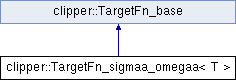Clipper
clipper::TargetFn_sigmaa_omegaa< T > Class Template Reference

`#include <resol_targetfn.h>`

Inheritance diagram for clipper::TargetFn_sigmaa_omegaa< T >:List of all members.

## Public Member Functions

TargetFn_sigmaa_omegaa (const HKL_data< T > &eo, const HKL_data< T > &ec)
constructor: takes the datalist against which to calc target
Rderiv rderiv (const HKL_info::HKL_reference_index &ih, const ftype &omegaa) const
return the value and derivatives of the target function

## Static Public Member Functions

static ftype sigmaa (const ftype &omegaa)
convert omegaa to sigmaa

## Detailed Description

### template<class T> class clipper::TargetFn_sigmaa_omegaa< T >

Deprecated:
simple sigma_a target function

This class implements the target function for calculating sigma_a. Required is a datalist containing Eo, Ec.

It actually refines omegaa = sigmaa/(1-sigmaa^2). This has better proerties for refinement. To get sigmaa use

``` sigmaa = ( sqrt( 4*omegaa^2 + 1 ) - 1 ) / ( 2*omegaa )
```

This is available as a static function:

``` sigmaa = targetfn.sigmaa( omegaa )
```

This version simplifies terms in |Eo|^2 and |Ec|^2 which should average out to 1 if the normalisation scheme is consistent with the sigmaa calc.

Convergence is good for calculations using the 'binner' basis function, however the smooth basis function have convergence problems. This is still under investigation.

The documentation for this class was generated from the following file: